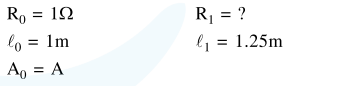# Solve this following

Question:

A wire of $1 \Omega$ has a length of $1 \mathrm{~m}$. It is stetched till its length increases by $25 \%$. The percentage change in resistance to the neartest integer is :-

1. $56 \%$

2. $25 \%$

3. $12.5 \%$

4. $76 \%$

Correct Option: 1

Solution:As volume of wire remains constant so

$\mathrm{A}_{0} \ell_{0}=\mathrm{A}_{1} \ell_{1} \Rightarrow \mathrm{A}_{1}=\frac{\ell_{0} \mathrm{~A}_{0}}{\ell_{1}}$

Now

$\operatorname{Resistance}(\mathrm{R})=\frac{\rho \ell}{\mathrm{A}}$

$\frac{\mathrm{R}_{0}}{\mathrm{R}_{1}}=\frac{\ell_{0} / \mathrm{A}_{0}}{\rho \ell_{1} / \mathrm{A}_{1}}$

$\frac{1}{\mathrm{R}_{1}}=\frac{\ell_{0}}{\mathrm{~A}_{0}}\left(\frac{\ell_{0} \mathrm{~A}_{0}}{\ell_{1} \times \ell_{1}}\right) \quad \mathrm{R}_{1}=\frac{\ell_{1}^{2}}{\ell_{0}^{2}}=1.5625 \Omega$

So $\%$ change in resistance

$=\frac{\mathrm{R}_{1}-\mathrm{R}_{0}}{\mathrm{R}_{0}} \times 100 \%$

$=\frac{1.5625-1}{1} \times 100 \%$

$=56.25 \%$